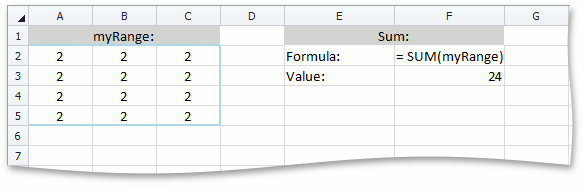21.1
20.2
20.1
19.2
19.1
18.2
18.1
17.2
A newer version of this page is available. Switch to the current version.

# How to: Use Names in Formulas

This example demonstrates how to use a named cell range in a formula. In some cases, it can be useful to name individual cells and cell ranges to make it easier to understand what information they contain.

##### NOTE

A complete sample project is available at https://github.com/DevExpress-Examples/winforms-spreadsheetcontrol-api-e4655

``````// Access the "A2:C5" range of cells in the worksheet.
Range range = worksheet.Range["A2:C5"];

// Specify the name for the created range.
range.Name = "myRange";

// Create a formula that sums up the values of all cells included in the specified named range.
worksheet.Cells["F3"].Formula = "= SUM(myRange)";
``````

The image below shows a named cell range, and the result returned by the formula using this range.See Also